### Home > CALC > Chapter 7 > Lesson 7.1.5 > Problem7-50

7-50.
1. Integrate. Homework Help ✎

1.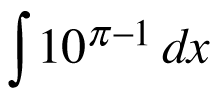2.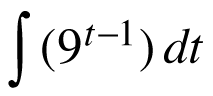3.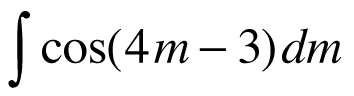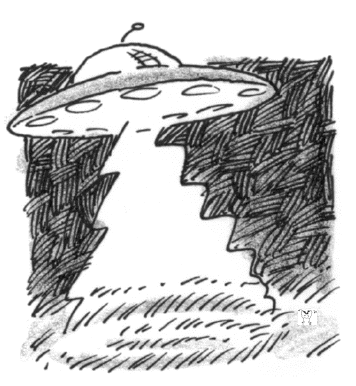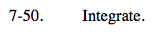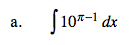What does the graph of y = 10π − 1 look like? What will its area function look like?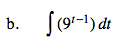$\text{Recall that }9^{t-1}=(9^{t})(9^{-1})=\frac{9^{t}}{9}.$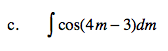= ksin(4m − 3) + C
Find the value of the constant k.